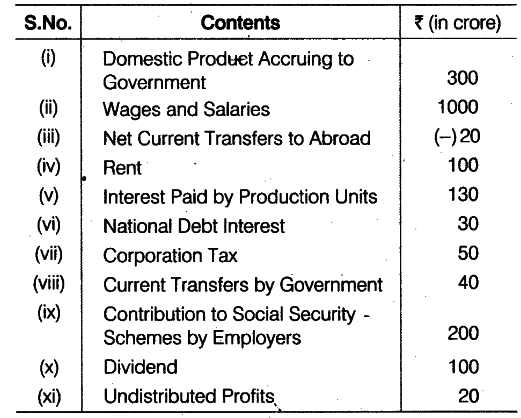# Calculate Private income from the following data:

Calculate (a) Net Domestic Product at Factor Cost and (b) Private income from the following data:(a) \$ND{{P}_{FC}}\$ = Wages and Salaries + Rent + Interest Paid by Production Units + Corporation Tax + Dividends + Undistributed Profits

• Contribution to Social Security Schemes by Employers
= 1000+100+130+50 +100+20+200 = Rs 1600 crore
(b) Private Income = \$ND{{P}_{FC}}\$ - Domestic Product Accruing to Government - Net Current Transfers to Abroad + National Debt Interest + Current Transfers by Government + Net Factor Income from Abroad
= 1600 - 300- (- 20)+ 30 + 40 + 0 = Rs 1390 crore